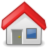## Syntax

# Syntax (new test)
# Less portable, works in modern shell like bash, ksh, zsh
if [[ condition ]]; then
# statements
fi

# Syntax (old test)
# Portable but painful
if [ condition ]; then
# statements
fi

~~~~~~~~~~~~~~~~~~~~~~~~~~~~~~~~~

## Example #1

#!/bin/bash
NAME=\$1                             # get the first argument
GREETING="Hi there"
HAT_TIP="*tip of the hat*"

if [ "\$NAME" = "Dave" ]; then
echo \$GREETING
elif [ "\$NAME" = "Steve" ]; then
echo \$HAT_TIP
else
fi

## Example #2 (with arguments)

#!/bin/bash
# file: gameoflife.sh

NUM_REQUIRED_ARGS=2

# Do we have at least 2 arguments?
if [[ \$# -lt \$NUM_REQUIRED_ARGS ]]; then
echo "Not enough arguments. Call this script with
,/{\$0} <name> <number>"

exit 1                          # exit with error
fi

# In terminal
./gameoflife.sh

# Output
Not enough arguments. Call this script with
./gameoflife.sh <name> <number>

## Example #3 (string comparison)

#!/bin/bash

str1="a"
str2="b"

# Equally (= and !=) (ASCII comparison)
if [ "\$str1" = "\$str2" ]; then
echo "\${str1} is equal to \${str2}!"
fi

if [ "\$str1" !"\$str2" ]; then
echo "\${str1} is not equal to \${str2}!"
fi

# Null (-n) or Zero length (-z)
not_nully="this is something (not nothing)"
nully=""

if [ -n "\$not_nully" ]; then
echo "This is not at all nully..."
fi

if [ -z "\$nully" ]; then
echo "nully/zeroooo (length)"
fi

# Output
a is not equal to b!
This is not at all nully...
nully/zeroooo (length)

## Example #4 (integer comparison)

#!/bin/bash

int1=1
int2=7

# Equal, not equal (-eq and -ne)
if [ \$int1 -eq \$int2 ]; then
echo "\${int1} is equal to \${int2}"
fi

if [ \$int1 -ne \$int2 ]; then
echo "\${int1} is not equal to \${int2}"
fi

# Greater than, less than +equal
# -gt -lt -ge -le
if [ \$int1 -gt \$int2 ]; then
echo "\${int1} is greater than \${\$int2}"
fi

if [ \$int1 -le \$int2 ]; then
echo "\${int1} is less than or equal to \${\$int2}"
fi

# String comparison operators can be used with double parenthesis
if (( \$int1 == \$int2 )); then
echo "\${int1} is equal to \${int2}."
fi

# (( ))
# ==    Is Equal to
# !=    Is Not Equal to
# <     Is Less than
# <=    Is Less than or equal to
# >     Is Greater than
#>=     Is Greater than or equal toIndex